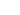# HLOOKUP Function

This article explains how to use HLOOKUP functions

# Sample Usage

``HLOOKUP(QA, DA, 1, 1)``
``HLOOKUP(135, DB, 2, 2)``

# Syntax

``HLOOKUP (value, datasheet, row_index, [range_lookup])``
• `value` - The value to look for in the first column of a datasheet.
• `datasheet` - The table from which to retrieve a value.
• `row_index` - The row in the datasheet from which to retrieve a value.
• `range_lookup` - [optional] 0 = exact match (default). 1 = approximate match.
• `range_lookup_higher_bound` - [optional] 0 = return lower value (default). 1 = return higher value
• Instead of a table range in a spreadsheet program (e.g. `A1:B22`), the value of the datasheet needs to be just the reference to that datasheet (eg. `DA`).